Get inspired by the success stories of our students in IIT JAM MS, ISI  MStat, CMI MSc DS.  Learn More

# Restricted Regression Problem | ISI MStat 2017 PSB Problem 7This problem is a regression problem, where we use the ordinary least square methods, to estimate the parameters in a restricted case scenario. This is ISI MStat 2017 PSB Problem 7.

## Problem

Consider independent observations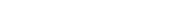from the regression model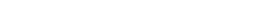where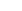andare scalar covariates,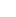and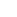are unknown scalar
coefficients, and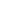are uncorrelated errors with mean 0 and variance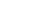. Instead of using the correct model, we obtain an estimate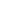ofby minimizing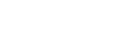Find the bias and mean squared error of.

### Prerequisites

• Ordinary Least Square Method
• Minimizing the Square Loss Error Function
• Multiple Regression
• Mean Square Error =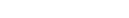.
• Bias

## Solution

It is sort of a restricted regression problem because maybe we have tested the fact that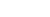. Hence, we are interested in the estimate ofgiven. This is essentially the statistical significance of this problem, and we will see how it turns out in the estimate of.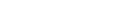Let's minimize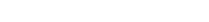by differentiating w.r.tand equating to 0.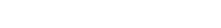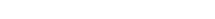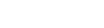From, the given conditions,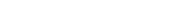.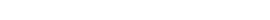.

Since,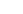are constant,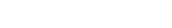.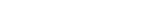.

Thus, observe that the moreis close to 0, the more bias is close to 0.

From, the given conditions,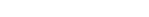~ Something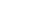).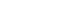~ Something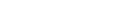.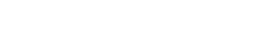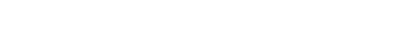Observe, that even the MSE is minimized if.

This problem is a regression problem, where we use the ordinary least square methods, to estimate the parameters in a restricted case scenario. This is ISI MStat 2017 PSB Problem 7.

## Problem

Consider independent observationsfrom the regression modelwhereandare scalar covariates,andare unknown scalar
coefficients, andare uncorrelated errors with mean 0 and variance. Instead of using the correct model, we obtain an estimateofby minimizingFind the bias and mean squared error of.

### Prerequisites

• Ordinary Least Square Method
• Minimizing the Square Loss Error Function
• Multiple Regression
• Mean Square Error =.
• Bias

## Solution

It is sort of a restricted regression problem because maybe we have tested the fact that. Hence, we are interested in the estimate ofgiven. This is essentially the statistical significance of this problem, and we will see how it turns out in the estimate of.Let's minimizeby differentiating w.r.tand equating to 0.From, the given conditions,..

Since,are constant,..

Thus, observe that the moreis close to 0, the more bias is close to 0.

From, the given conditions,~ Something).~ Something.Observe, that even the MSE is minimized if.

This site uses Akismet to reduce spam. Learn how your comment data is processed.

### 6 comments on “Restricted Regression Problem | ISI MStat 2017 PSB Problem 7”

1.SOMJIT ROY says:

I guess the estimate of Beta 1 here is slightly incorrect .

2.akshay says:

beta 1 estimate is wrong dude !!!

3.akshay says:

beta 1 estimate is wrong dude.

4.Giorno Giovana says:

Mistake in taking derivative..Whole solution gone wrong..Yare yare daze

5.Giorno Giovana says:

Mistake in taking derivative. Whole solution gone wrong..

6.Rajeev Kumar says:

Yes srijit da!!
Estimate value of β1 is not true

### Knowledge Partner